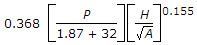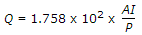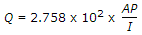# Civil Engineering - Water Supply Engineering

### Exercise :: Water Supply Engineering - Section 2

26.

During treatment of water, sedimentation is done

 A. before filtration B. after filtration C. simultaneously with filtration D. along with chlorination.

Explanation:

No answer description available for this question. Let us discuss.

27.

E. Coli bacterias die in water having pH greater than

 A. 5.5 B. 6.5 C. 7.5 D. 8.5 E. 9.5

Explanation:

No answer description available for this question. Let us discuss.

28.

Check valves are installed

 A. on the delivery side of the pumping set B. at the interconnections between polluted water system and a potable water system C. both (a) and (b) D. neither (a) nor (b).

Explanation:

No answer description available for this question. Let us discuss.

29.

The equationin which Q is yearly run off in cm, P is yearly total rainfall in cm, H is difference of R.L.s. of lowest and highest points and A is area of catchment in square metres, is known as

 A. English formula B. Khosla's formula C. Justin's formula D. Vermule's formula.

Explanation:

No answer description available for this question. Let us discuss.

30.

If intensity of rainfall in cm per hour is I, percentage coefficient of run-off is P, area of catchment in square kilometres is A, the total run-off Q, is given by

 A. Q = 1.758 x 102 x API B. Q = 2.758 x 102 x API C. Q = 2.758 x 103 x API D.E.